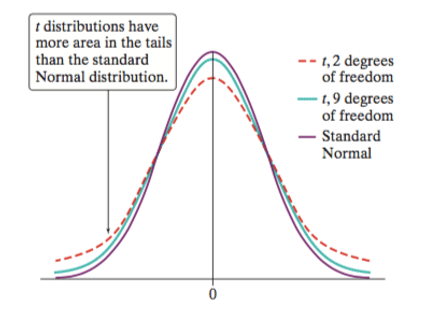## Day 84 - Lesson 8.3

##### Learning Targets
• Determine the critical value for calculating a C% confidence interval for a population mean using a table or technology.

• State and check the Random, 10%, and Normal/ Large Sample conditions for constructing a confidence interval for a population mean.

• Construct and interpret a confidence interval for a population mean.

##### Activity:

Nabisco, the company that owns Oreo, claims that the true mean weight of an Oreo cookie is 11.3 grams. In this Activity, students will be using sample data to examine the potential evidence against this claim. You might want to have 30 Oreos in the front of the classroom to make the data more realistic – actually this is just so that each student leaves class with an Oreo. I promise this will help when you are recruiting students for next year’s AP Stats class.

##### Why t* instead of z*?

Too many students are conditioned to memorize “z* for proportions, t* for means“. While this is true, this statement does not answer the why. The short answer is that when trying to estimate the mean of a population, we also have to estimate the standard deviation of the population in order to understand the standardized distribution of sample means. Because we are now trying to estimate 2 parameters, we are introducing more variation into the standardized statistic. Thus the distribution of sample means follows a t-distribution, which is wider and shorter than a Normal distribution. We will give a more detailed explanation in Section 9.3.##### How to satisfy the Normal/Large Sample condition?

There are three potential ways to satisfy the Normal/Large Sample Condition

Easy: If the population distribution is (approximately) Normal, then the sampling distribution of the sample mean is (approximately) Normal.

Medium: If the population distribution is not Normal (or shape is unknown), the sampling distribution of the sample mean is approximately Normal if the sample size is large enough, which is 30 in most cases. Students learned about the Central Limit Theorem in Section 7.3.

Hard: If the shape of the population is unknown and the sample size is small, graph the sample data. If the sample data shows no strong skewness and no outliers, it is reasonable to assume the population distribution is approximately Normal (meaning the sampling distribution is approximately Normal).

##### Inferential Thinking

When we get to Chapter 9, Nabisco’s claim that the mean weight of an Oreo is 11.3 grams will become the null hypothesis. Students will then use the data to determine whether or not we have convincing evidence against this claim by calculating a P-value. We can get students already thinking about this by asking whether or not the confidence interval provides convincing evidence against the claim (it does!).